# Comparing Fractions: Lesson for Kids

Instructor: Jessica Lowe
How do you figure out if you'd rather have 1/2 or 2/3 of a sandwich for lunch? This lesson uses real world examples and pictures to help you understand how to compare fractions.

## What is a Fraction?

Your best friend has a candy bar in their lunch. Because you share everything, they ask if you want 1/2 or 3/5 it. Now, you want the most chocolate you can get, right? Follow along to learn how to figure this out.

Before we get started, let's review what a fraction is. A fraction is a number less than one. This model shows the fraction 3/5.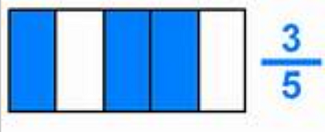The 5 is the number of equal pieces the model is broken into. This is called the denominator. The 3 is the number of parts we're talking about. This is called the numerator. It's what is colored blue.

The numerator is the number on top of the fraction line, and the denominator is the number underneath the fraction line. See picture A.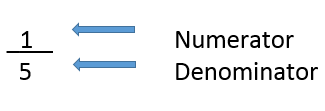## Comparing Fractions

Now, that we've reviewed the parts of a fraction, let's get back to the chocolate. How do you figure out if 1/2 or 3/5 is bigger?

### Steps:

1. Write the fractions side by side, leaving a little room in the middle for the less than, greater than, or equal to sign (more about those later).

2. Multiply the numerator in the first fraction, which is 1, by the denominator in the second fraction, which is 5. Write the answer, which is 5, next to the numerator in the first fraction (1 x 5 = 5).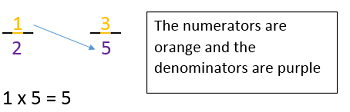3. Multiply the numerator of the second fraction, which is 3, times the denominator in the first fraction, which is 2. Write the answer, which is 6, next to the numerator in the second fraction (3 x 2 = 6).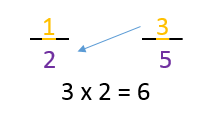4. Which number next to the numerators is greater? The 6 next to the second fraction is greater, so the second fraction is larger. It's that simple!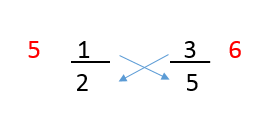You tell your best friend that you want 3/5 of the chocolate bar.

### Writing it Out

When comparing fractions, you use the 'less than', 'greater (bigger) than', and 'equal to' signs. See picture B.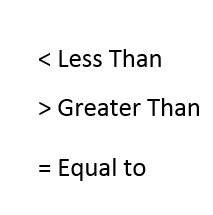5. So, going back to the chocolate example, you'll write the 'less than' sign in the middle since 1/2 is less than 3/5. See picture C.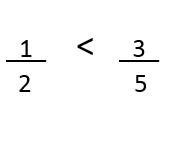To unlock this lesson you must be a Study.com Member.

### Register to view this lesson

Are you a student or a teacher?

#### See for yourself why 30 million people use Study.com

##### Become a Study.com member and start learning now.
Back
What teachers are saying about Study.com

### Earning College Credit

Did you know… We have over 200 college courses that prepare you to earn credit by exam that is accepted by over 1,500 colleges and universities. You can test out of the first two years of college and save thousands off your degree. Anyone can earn credit-by-exam regardless of age or education level.